# FER-2013

The Facial Expression Recognition 2013 (FER-2013) Dataset

Originator: Pierre-Luc Carrier and Aaron Courville

Classify facial expressions from 35,685 examples of 48x48 pixel grayscale images of faces. Images are categorized based on the emotion shown in the facial expressions (happiness, neutral, sadness, anger, surprise, disgust, fear).

## Examples

### Basic Examples

Retrieve the resource:

 In:=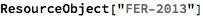Out=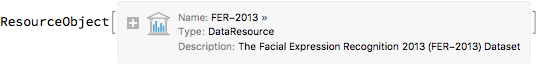Retrieve a sample of the data:

 In:=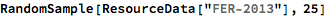Out=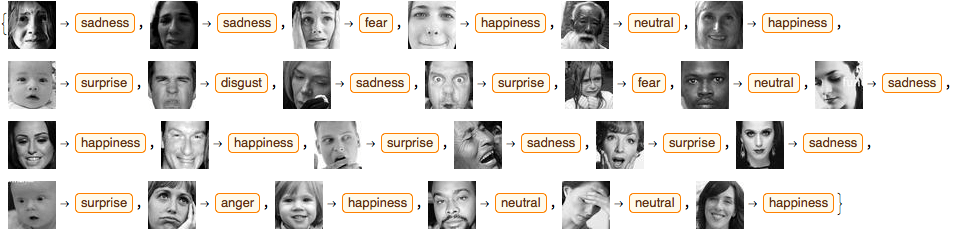### Analysis

Select one image of each facial expression:

 In:=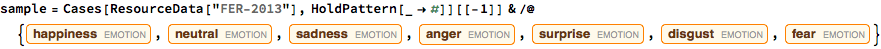Out=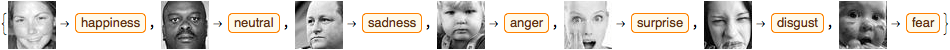Use a built-in classifier:

 In:=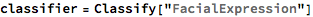Out=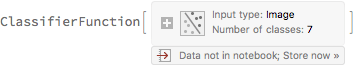Test the built-in classifier on a set of images:

 In:=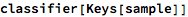Out=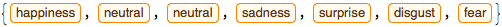Create a larger test set:

 In:=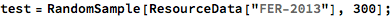Measure the accuracy of the classifier on the test set:

 In:=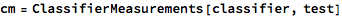Out=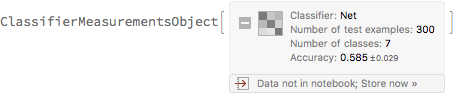Visualize that accuracy of the classifier:

 In:=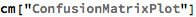Out=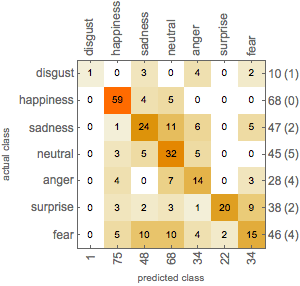Wolfram Research, "FER-2013" from the Wolfram Data Repository (2018)